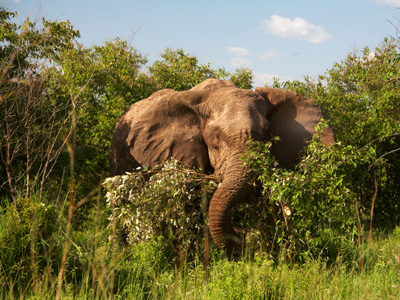An African elephant exerts pressure on the ground on which it stands. That pressure is measured in pascals.

# Pressure 01

## A fascinating way to revise about forces, newtons (N) and pascals (Pa). Who was Pascal?

### Scroll down to play the quiz

This KS3 Science quiz asks questions about pressure. Pressure is an effect which occurs when a force is applied on a surface. It is the amount of force acting on a unit area, so in order to calculate pressure, we need to know the force applied and the area it is acting on. The SI unit for force is the newton (N), named after the famous scientist of the same name - Isaac Newton. If the area is given in square metres (m2) and the force acting is given in newtons, then the units of pressure will be pascals (Pa).

The pascal is named after the French mathematician and scientist Blaise Pascal. He was particularly interested in fluids and pressure and invented the hydraulic press. This device uses pressure to magnify forces. At the time Pascal was alive, most scientists refused to believe in the concept of a vacuum. It was Pascal who demonstrated that a vacuum really existed. It was in honour of his work on pressure that the unit of pressure was named after him.

1.
What is force measured in?
Joules
Kilograms
Newtons
Pascals
Although the symbol for force is a capital N, when writing it down, unless it is at the start of a sentence, it is written as newton, without a capital letter
2.
What are the SI units of pressure?
Joules
Kilograms
Newtons
Pascals
1 Pa = 1 N / 1 m2
3.
If pressure is measured in pascals, Pa, and force in newtons, N, then area is measured in .......
cm2
km2
m2
mm2
You always need to be careful about which units you use
4.
If pressure increases then .......
force and area are doubled
force and area are halved
force increases or area decreases
force increases or area increases
This is because pressure = force/area
5.
A box weighs 100 N. The area of the box on the ground is 20 m2. What is the pressure of the box on the ground?
0.02 Pa
5 Pa
50 Pa
2,000 Pa
Pressure = force/area = 100 N/20 m2 = 5 Pa
6.
A box weighs 60 N. The dimensions of the base are 3 m by 2 m. What is the pressure of the box on the ground?
10 Pa
12 Pa
20 Pa
30 Pa
Pressure = force/area, area = 3 m x 2 m = 6 m2, pressure = 60/6 = 10 Pa
7.
A sheet of metal in a factory weighs 60 N. Its dimensions are 2 m by 1 m. What is the pressure it puts on the ground?
6 Pa
20 Pa
30 Pa
60 Pa
Area of metal sheet = 2 m x 1 m = 2 m2 so pressure = 60/2 = 30 Pa
8.
A student weighs 600 N. The area of one foot is 0.01 m2. If she stands on two feet what is the pressure on the ground?
300 Pa
6,000 Pa
30,000 Pa
60,000 Pa
Area of two feet = 0.01 x 2 = 0.02 pressure = 600/0.02
9.
The dimensions of the base of a box are 80 cm by 100 cm. It weighs 400 N. What is the pressure on the ground?
50 Pa
80 Pa
400 Pa
500 Pa
80 cm = 0.8 m, 100 cm = 1 m, area = 0.8 x 1 = 0.8 m2, pressure = 400/0.8 = 500 Pa
10.
The size of an African elephant's foot is 40 cm by 50 cm. The elephant weighs 64,000 N. When standing on the ground, what is the pressure it exerts?
40,000 Pa
80,000 Pa
240,000 Pa
300,000 Pa
Area of foot = 40 cm x 50 cm = 0.4 m x 0.5 m = 0.2 m2, area of four feet = 0.8 m2, pressure = 64,000/0.8